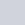If one of the two electrons of a
Question:

If one of the two electrons of a Hmolecule is removed, we get a hydrogen molecular ion. In the ground state of an, the two protons are separated by roughly 1.5 Å, and the electron is roughly 1 Å from each proton. Determine the potential energy of the system. Specify your choice of the zero of potential energy.

Solution:

The system of two protons and one electron is represented in the given figure.Charge on proton $1, q_{1}=1.6 \times 10^{-19} \mathrm{C}$

Charge on proton 2, q2 = 1.6 ×10−19 C

Charge on electron, q3 = −1.6 ×10−19 C

Distance between protons 1 and 2, d1 = 1.5 ×10−10 m

Distance between proton 1 and electron, d2 = 1 ×10−10 m

Distance between proton 2 and electron, d3 = 1 × 10−10 m

The potential energy at infinity is zero.

Potential energy of the system,

$V=\frac{q_{1} q_{2}}{4 \pi \epsilon_{0} d_{1}}+\frac{q_{2} q_{3}}{4 \pi \epsilon_{0} d_{3}}+\frac{q_{3} q_{1}}{4 \pi \epsilon_{0} d_{2}}$

Substituting $\frac{1}{4 \pi \epsilon_{0}}=9 \times 10^{9} \mathrm{Nm}^{2} \mathrm{C}^{-2}$, we obtain

$V=\frac{9 \times 10^{9} \times 10^{-19} \times 10^{-19}}{10^{-10}}\left[-(16)^{2}+\frac{(1.6)^{2}}{1.5}+-(1.6)^{2}\right]$

$=-30.7 \times 10^{-19} \mathrm{~J}$

$=-19.2 \mathrm{eV}$

Therefore, the potential energy of the system is $-19.2 \mathrm{eV}$.